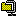## Interoperable string encrypters for the web.

2007. 12. 19. 16:01StringEncrypter.zip

A new version is available for ASP and ASP.NET.

I've written string encrypters for ASP, ASP.NET 1.1/2.0, JSP and PHP 5 respectivly, which are interoperable with each other.
The encrypters take two strings, a key and an initial vector. The key and the initial vector are internally converted into UTF-8 and hashed by MD5.String encryption is carried out by using Encrypt method, which first converts the input string into UTF-8 and encodes it according to the PKCS7 padding algorithm (except JSP that uses PKCS5, but it is compatible if the block size is 128bit.) and encrypts it with 128-bit AES algorithm in Cipher Block Chaining(CBC). The encrypted binary is encoded by Base64.
Decrypt method decrypts the encrypted string.The following code is an example of using the string encrypter in ASP.NET 1.1/2.0(C#). The StringEncrypter class is implemented in StringEncrypter.cs.
```/*
*  ASP.NET 1.1/2.0 (C#)
*/
using Hyeongryeol.Security.Cryptography ;
...
const string key = "This is a secret key." ;
const string iv = "This is an initial vector." ;

// Create an instance.
StringEncrypter encrypter = new StringEncrypter (key, iv) ;

// Encrypt a string.
string encrypted = encrypter.Encrypt ("Test sample") ;

// Decrypt a string.
string decrypted = encrypter.Decrypt (encrypted) ;
```
The following code shows how to use the string encrypter in ASP(VBScript). Since ASP doesn't support built-in AES algorithm, you need to install HyeongryeolStringEncrypter.dll which is a COM component based on XySSL 0.8. You have to register it by using the regsvr32.exe program.
(I recommend you to install it in the %WINDOWS%System32 folder.)
```'
'  ASP (VBScript)
'
Const conKey = "This is a secret key."
Const conIV = "This is an initial vector."

' Create an instance.
Set objEncrypter = Server.CreateObject ("Hyeongryeol.StringEncrypter")

objEncrypter.Key = conKey
objEncrypter.InitialVector = conIV

' Encrypt a string.
strEncrypted = objEncrypter.Encrypt ("Test sample")

' Decrypt a string.
strDecrypted = objEncrypter.Decrypt (strEncrypted)
```
The following code is an example in JSP (Java). The StringEncrypter class is implemented in StringEncrypter.java which also requires Base64Encoder.java.
```/*
*  JSP (Java)
*/
import com.hyeongryeol.security.cryptography.*;
...
String key = "This is a secret key.";
String iv = "This is an initial vector.";

// Create an instance.
StringEncrypter encrypter = new StringEncrypter(key, iv);

// Encrypt a string.
String encrypted = encrypter.encrypt("Test sample");

// Decrypt a string.
String decrypted = encrypter.decrypt(encrypted);
```
The following code is an example in PHP 5. The StringEncrypter class is implemented in StringEncrypter.php. It can only be used in a UTF-8 encoded file because UTF-8 encoding and decoding are omitted.
```/*
*  PHP 5 (UTF-8)
*/
require ("StringEncrypter.php") ;

define ("KEY", "This is a secret key.") ;
define ("IV", "This is an initial vector.") ;

// Create an instance.
\$encrypter = new StringEncrypter (KEY, IV) ;

// Encrypt a string.
\$encrypted = \$encrypter->encrypt ("Test sample") ;

// Decrypt a string.
\$decrypted = \$encrypter->decrypt (\$encrypted) ;
```

References
YOUR COMMENT IS THE CRITICAL SUCCESS FACTOR FOR THE QUALITY OF BLOG POST
1. 라이언 2014.12.01 13:13

감사히 잘 사용하고 있습니다.
그런데 이번에 서버를 업그레이드하면서 64비트로 바꿨더니 등록이 안되네요.
64bit 에 맡게 변경하려면 어떻게 해야하나요?
이것저것 해보고 있는데 어렵네요.

부탁드립니다.

2. 64비트안됨 2016.04.05 17:54

안됩니다.